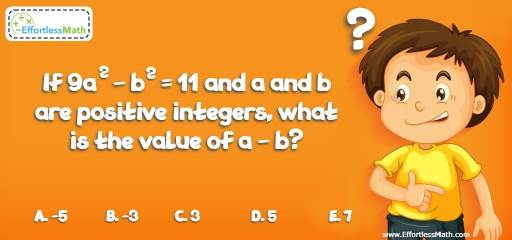# Algebra Puzzle – Challenge 54

This is a great math challenge for those who enjoy solving math and algebra challenges! The solution is also given.## Challenge:

If $$9a^2 – b^2 = 11$$ and a and b are positive integers, what is the value of $$a – b$$?

A- $$-5$$

B- $$-3$$

C- 3

D- 5

E- 7

### The Absolute Best Book to challenge your Smart Student!

Factorize $$9a^2 – b^2$$ and 11
(3a – b) (3a + b) = 11 × 1
Therefore, 3a – b equals to 11 or 1 and 3a + b equals to 1 or 11.
Let’s check both:
3a – b = 1 and 3a + b = 11
Solve the above system of equation:
a = 2 and b = 5
3a – b = 11 and 3a + b = 1
a = 2 and b = – 5
Since, a and b are positive integers, then, only a = 2 and b = 5 are the solutions.
Therefore, a – b = 2 – 5 = -3

The Best Books to Ace Algebra

### What people say about "Algebra Puzzle – Challenge 54 - Effortless Math: We Help Students Learn to LOVE Mathematics"?

No one replied yet.

X
45% OFF

Limited time only!

Save Over 45%

SAVE $40 It was$89.99 now it is \$49.99